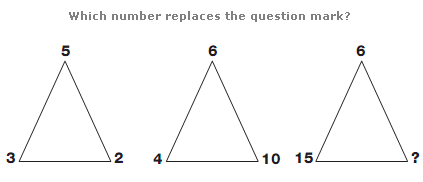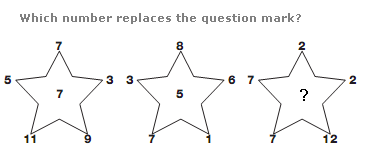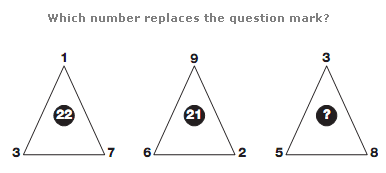# Puzzles - Number puzzles

### Exercise :: Number puzzlesAnswer : 9 Explanation : Working from left to right, the sum of the two smaller numbers on each triangle equals the third number.Answer : 6 Explanation : The number in the centre of each star equals the average of the surrounding 5 numbers.Answer : 43 Explanation : In each triangle, multiply the lower two numbers together and add the upper number to give the value at the centre.